# Team:SYSU-Software/model

Team:SYSU-Software new

# Models and Algorithms

## Overview

Mathematical modeling is the soul of our magical software "Computer Aided Synbio Tools", CAST. In our models, we take into account the intrinsic dynamics of different circuits or systems hierarchically by deterministic, stochastic and time delay models. Here are the highlights of our models and algorithms:

* We place great emphasis on various kinds of promoters, operons, coding parts, RBSs, terminators and combine regulatory as well as metabolic ODEs and Hill equations in our multi-level modeling;

* We create a new iteration and optimization algorithm to calculate the fold-change in different cascades and cyclic circuits to evaluate the strength of various regulators;

* Leakage rate diversity and copy number of different plasmids are considered for better reflection of complex biological systems and experimental results in vivo;

* What our modeling tries to achieve is to creatively build a bridge between parts and modules in the Registry and widespread experimental data in the papers so that researchers can have a brand new perspective of how these parts, sequences and kinetic parameters are related to each other;

* Standardized output PoPS, RIPS is generated from our data processing and testing procedures, which represents our goal of establishing sets of standardized data;

* As the current focus of synthetic biology is readily combining modules into complex synthetic pathways, statistical and probability distribution models are also applied to estimate extrinsic variability among synthetic systems.

## Intrinsic dynamics

### Parameters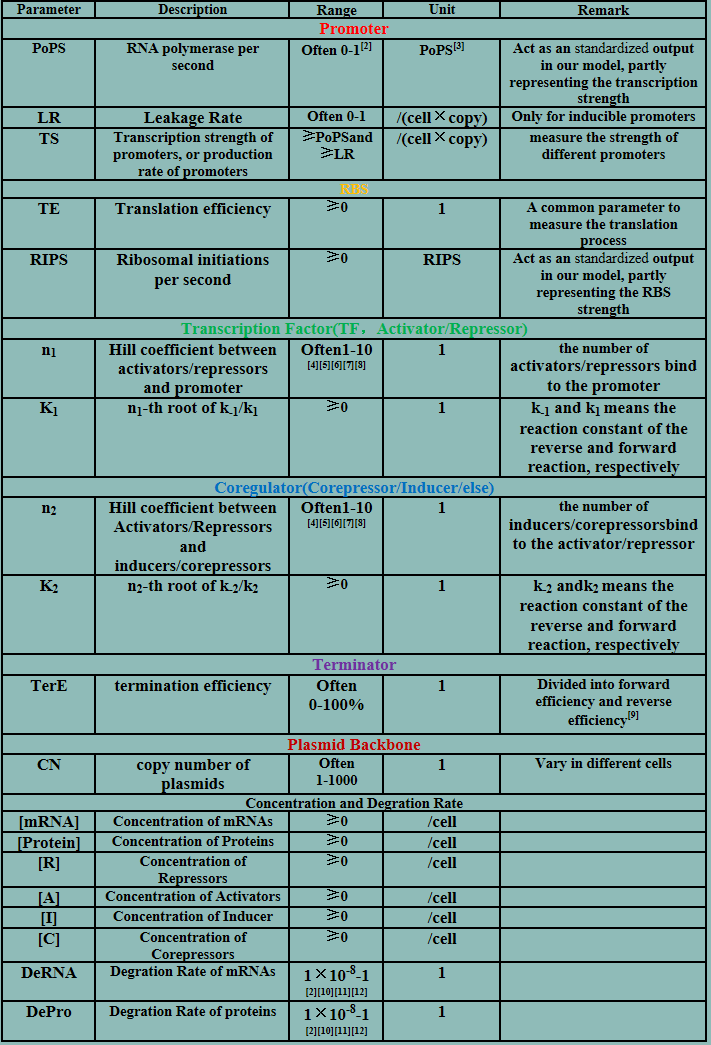## Deterministic models

The model mainly emphasizes on leakage rate of different promoters, the mRNA degradation term, and the strength of transcription, or called the rate of production. Also, transcription factors, such as repressors and activators, and coregulators are introduced to describe the dynamics of operons. In addition, the target DNA sequence is placed on Copynumber (CN) plasmids and some parameters are defined, for example, TS means the transcription strength of promoters while DeRNA represent the degradation rate of mRNA. Besides, we apply different levels of Hill input functions to model the behaviors of these regulators.

### 1. Constitutive Promoter

Transcription: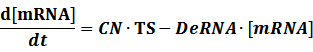Translation: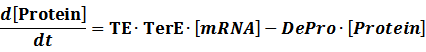(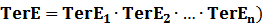According to the iGEM Registry, the standardized input and output PoPS and RIPS can be given by the equations below based on our models: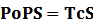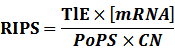At steady level, the concentration of mRNA and Protein do not change.

Therefore, we have: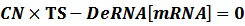Thus,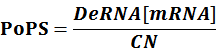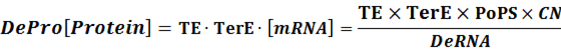When there is a standardized output, we have: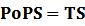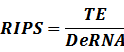### 2. Inducible Promoter

#### 2.1 one operon, positive or negative inducible

The first level: no transcription factor is added.

Here, we would introduce the leakage rate in order to estimate the production rate of the inducible promoters when no repressor or activator binds to the operon. Please note that, in negative inducible promoter, the leakage rate (LR) is almost as same as the transcription strength(TS) of the promoter in this situation.

Negative:Positive: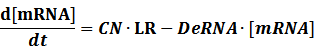The second level : Promoter+n1 repressors or activators

##### (1) Negative inducible promoter

In repression of a promoter, n repressor proteins, and nR, bind together to the operator, a DNA site of the promoter, and thus there is a decrease in the rate of transcription of the promoter, so we have the basic kinetic equations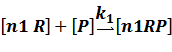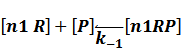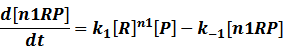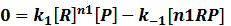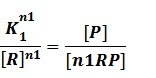where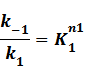Suppose that the whole promoter regions,[PT], consist of active regions and inactive regions while there are two repressors, both active and inactive, can blind with regions, transcription can be only occurred in active regions.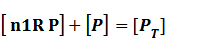Thus, we could conclude that the promoter activity can be defined as (exclude the leakage part)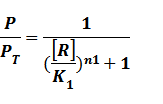And we can find the ODE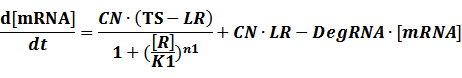##### (2) Positive inducible promoter>

When the specific region is bound to n1 activators,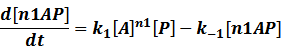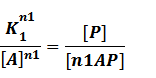In our model, the difference between positive and negative regulation is that positive regulated circuits is for the regions that blind with active activators and can perform transcription.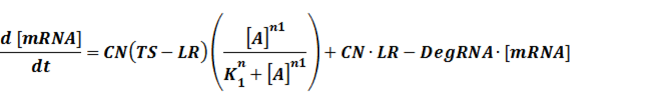The third level: Promoter+n1 repressors or activators+n2 inducers or corepressors.

There are four cases at this level:

##### (1) Repressors + Inducers: every repressor is inactivated by n inducers;

Similarly,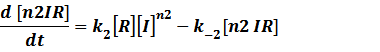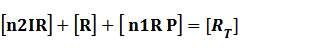Because [n1RP] << [n2IR]+[R], we can apply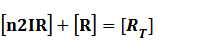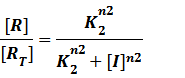AndAt last we can deduce that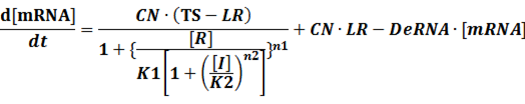##### (2) Repressors + Corepressors: every repressor is activated by n corepressors;

Similarly,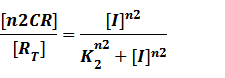We assume that [n2CR] takes part in the repression of the promoter. Therefore, we have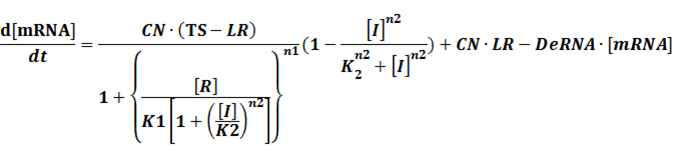To simplify it,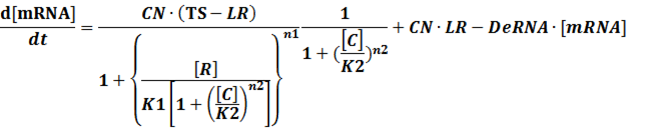##### (3) Activators + Corepressors: every activator is inactivated by n corepressors;

Like (1), we can deduce that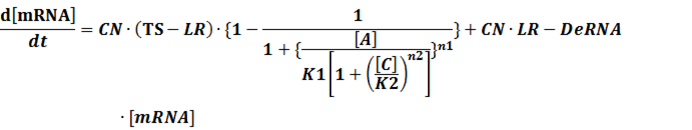##### (4) Activators + Inducers: every activator is activated by n inducers;

We assume that [n2IR] also takes part in the activation of the promoter, so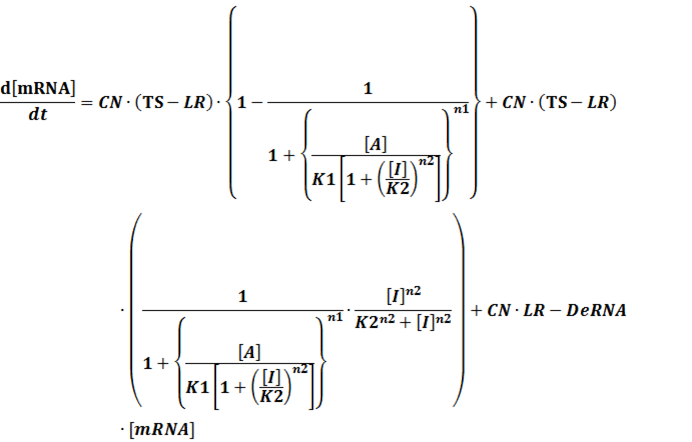To simplify it,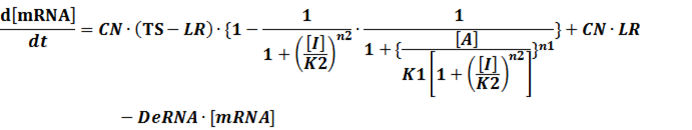In conclusion, the output PoPS in these four cases can be defined as: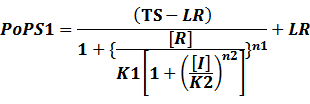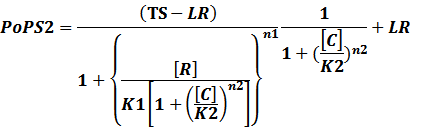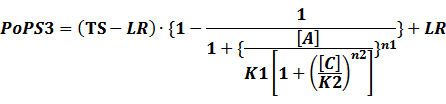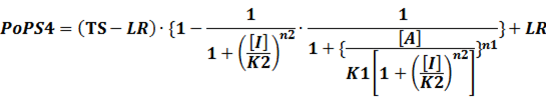Note that when there is no inducer or corepressor, [C] and [I] would be 0. However, the standardized output RIPS always remains the same. Thus, we have#### 2.2 two operons O1 and O2 (double promoter)

It is common for prokaryotic cells to have two operons in a specific DNA site and there are also many useful double promoters in the iGEM Registry database. For example, BBa_I739104 is a double promoter (LuxR/HSL, positive / P22 cII, negative). In order to model these circuits, several rules and situations have to be considered:

(1)If both O1 and O2 host repressors, they can bind either cooperatively or not. Cooperativity between repressors implies an increase of the repressor affinity towards O2 when O1 is occupied;

(2)If both O1 and O2 host activators, they can bind either cooperatively or give rise to synergistic activation, which increases the promoter strength with a consequent increment of the transcription initiation frequency;

(3)O1 hosts an activator, while O2 hosts a repressor, which has proved to be easier for oscillator-building;

(4)Just to be simple, we do not consider the complete competition and cooperativity among the inducers or corepressors.

Like the example BBa_I739104 mentioned above, we can deduce the following equation for the transcription process: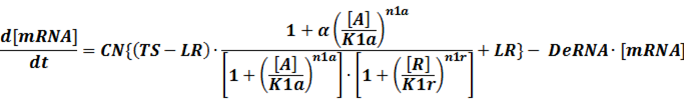When α is the fold-change of activated transcription, n1a and n1r means the hill coefficient of the activator and repressor, respectively.

### 3.Metabolic network

There are also cases where metabolic networks like enzymatic reactions and degradation process are involved. And metabolic reactions are also suitable for the transition from monomeric molecules to polymers like the mature of repressor protein. In these cases, we apply refined Michaelis-Menten equations to describe them.

Case I: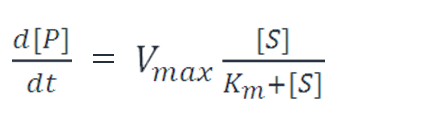CaseⅡ: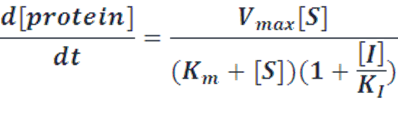Case Ⅲ: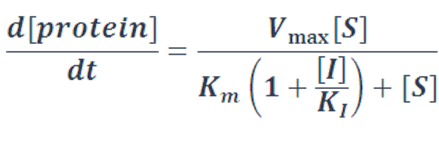## Stochastic and Time delay models

### 1.Stochastic models

Stochasticity in synthetic networks arises from fluctuationsin transcription and translation.First, the binding among different parts are the result of random encounters betweenmolecules, some of which are present in small numbers; What’s more, the time evolution of a biological reacting system is not a continuous process because molecular population levels can only change by discrete integer amounts. As a result, stochastic models are introduced to describe the dynamics of single molecules.

The most famous and common model is Gillespie Algorithm, but the obvious disadvantages of this algorithm is that a lot of calculations has to be performed. Due to the efficiency of our software, we adopt a Poisson tau-leaping algorithmas following:

1.Choose a time step,τ, we recommend0.1s as a time step.

2.Multiply each term of each differential equation( the right-hand values) by τ.

3. Apply Poisson distribution function to each result from step 2 and generate a random number using the Poisson distribution.

4. Calculate the new number of molecules by adding or subtracting each random number from step 3, from the initial number of molecules.

5. Repeat steps 1-4 for a specified number of reactions, N.

To make it more stable for calculation, we divided the right side of our deterministic equations into synthetic parts and degradation parts, for example,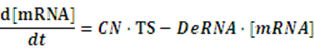CN∙TS is the synthetic part,DeRNA∙ [mRNA] is the degradation part. For each part, we generate Poisson random numbers and the other procedures remain the same. We find it more aligned with the real biological situations.

2. time delay models

Knowledge of molecular noise filtering and biochemical process delay compensation in synthetic circuits is crucial to understand the design of noise-tolerantand delay-robust gene circuits for synthetic biology.A linear stochastic time-delayed model is applied in our software to mimic the realistic dynamic behavior of anetwork.Where mRNA(t)=[mRNA1(t),…,mRNAn(t)]T, α0and αk denote the real-time and delay-time interactionamong these circuits, respectively, while the delay time τk rests on the specific circuits.

Cascades including tandem and cyclic ones are widely used in synthetic biology for different purposes. In order to get a better understanding of these circuits, we create a new iteration and optimization algorithm to calculate the fold-change in different cascades. Here is our story:

To begin with,a set of differential equations that represents the character of regulatory network generate an equilibrium solution, which means that the concentration of mRNA and the concentration of protein in the system wouldtend tohave fixed values after periods of time. Therefore, we conclude that the equilibrium solution and the initial conditions in this model are independent to each other and thus the equilibrium solution could be applied to represent the expression level of related circuits.

The equilibrium solution is also applied to describe the function of activators, repressors, corepressors and inducers at a regulation rate, which is also known as fold-change, between before regulation and after regulation.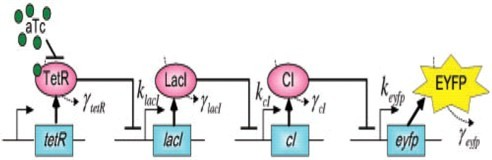### The First Level

If any activator or repressor does not bind to the operon, we have the set of differential equations below: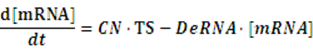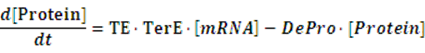As the initial concentration of mRNA and the initial concentration of protein have no effect on the equilibrium state, we define the initial values of both concentrations as follow: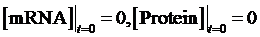Then, we have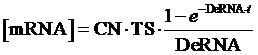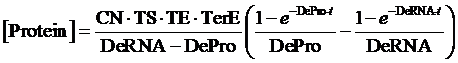Therefore, the equilibrium concentration of the targeted portion can be given by the following formula:

(1)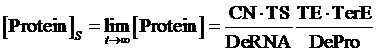In the equilibrium state, there is no rate of change in any variable so the above equilibrium concentration can also be given by the following set of equations: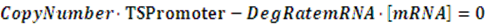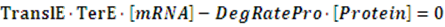Similarly, if the promoter is a positive induced promoter and there is no activator, the equilibrium concentration of the targeted protein is given by:

(2)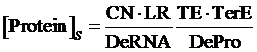As same as the above, if the promoter is a negative induced promoter and no repressor is presented, the equilibrium concentration of the targeted protein is given by:

(3)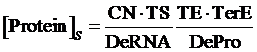### The Regulation Rate of Activator and Repressor

At first, we consider the computation of the regulation rate of activator.If the promoter is a positive regulated promoter and the promoter is regulated by an activator, we have the following set of differential equations: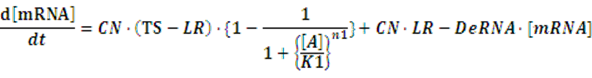The concentration of activator is a parameter, which would change its value with time. However, with respect to equilibrium state, it is meaningless to consider the change in the concentration of activator. The more important we concerned is the equilibrium concentration. Therefore, we would let the concentration of activator be aconstant,or, but the set of differential equations is still complicated for solving. As what has been done above, we assume there is no rate of change in any concentration when the system is in equilibrium so we can get the following set of equation: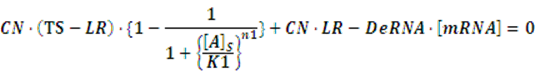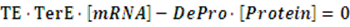Thus, the equilibrium concentration of targeted protein is:

(4)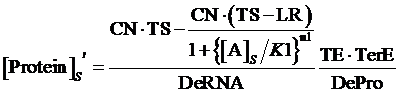Also, we understand that when the promoter is not regulated by activator, its equilibrium concentration of targeted protein should be:Therefore, the regulation rate of activator is:

(5)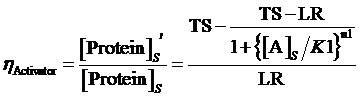Similarly, if the promoter is controlled by a negative induced promoter and regulated by repressor, the equilibrium concentration is given by:

(6)and the regulation rate of repressor is given by:

(7)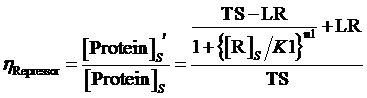where means the equilibrium concentration of repressor for the regulation of negative expressive induced promoter in such gene.

The Regulation Rate of Corepressor and Inducer

Applying the same method mention above, we can still find out the situation of targeted protein in the existence of activator or repressor with corepressor or inducer.

If the promoter is controlled by an activator, which regulated by a inducer and a co-repressor, the equilibrium concentration of targeted protein can be given by:

(8)While, same situation applies but, at this time, the promoter is regulated by an inducer instead of a corepressor, the equilibrium concentration of targeted protein can be given by:

(9)If the promoter is controlled by a repressor and regulated by a repressor and an inducer, the equilibrium concentration of targeted protein can be given by:

(10)Similarly, for corepressor regulation instead of inducer regulation, the equilibrium concentration of targeted protein can be given by:

(11)Moreover, based on the computation of equilibrium concentration of targeted protein with no regulation mentioned above, we can further have the following four equations regarding the regulatory rate in the presence of corepressor and inducer:

(12)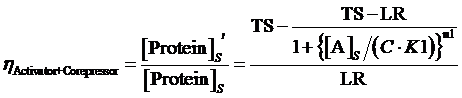，where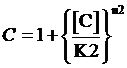(13)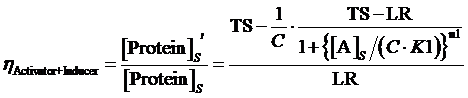，where(14)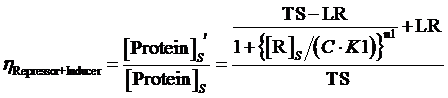，where(15)，where### Computation of Regulation Rate in synthetic cascades

The limitation of the above equation derivation is that we assume the equilibrium concentration of activator and repressor are known values but, in a real regulatory network, there are some factors would affect those equilibrium concentrations due to the fact that activator and repressor are parts of the targeted proteins in such network. Therefore, we cannot just simply apply (1)(2)(3)to compute its equilibrium concentration and find out the related regulation rates by applying (5)(7)(12)(13)(14)(15). In the following passage, we would explore how to compute different regulation rates in a gene more effectively with respect to a general regulatory network. And we would use to represent the regulation rate.

Given the network would arrange some circuits, Circuit1,Circuit2,...,CircuitN, in an order. The expression of the first gene is not regulated while the genes behind are regulated by formers regulatory proteins, i.e. acts as an activator or a repressor on. The algorithm of regulation rate is as follow.

#### Algorithm 1(tandem circuits)

Step 1: To compute the equilibrium concentrations of targeted proteins,[Protein1]s,[Protein2]s,...,[ProteinN]s, in existence of no regulation based on equation (1)(2)(3)with respect to the type of promoter.

Step2: As the expression of is not regulated, the equilibrium concentration of its target protein is [Protein1]s'=[Protein1]s

Step 3: There is a cycle for the remaining Circuitk(k=2,...,N). Based on the type of actual regulation, we substitute Circuitk-1’s equilibrium concentration of targeted protein obtained from the former cycle into one of the following equations, (4)(6)(8)(9)(10)(11). Then, we can get ’s equilibrium concentration of targeted protein,[Proteink]s' .

Step 4: To compute the regulation rate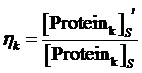according to the values of [Protein1]s,[Protein2]s,...,[ProteinN]s obtained in step 1 and the values of [Protein1]s',[Protein2]s',...,[ProteinN]s' obtained in step 2.

Algorithm 1 can only be applied effectivelyto chain regulatory network but, in a real situation, we may need to deal with cyclic regulatory network.

In cyclic regulatory network, every targeted protein shown on the cyclic path is responsible for one expression of the genes so it does not have such entry in algorithm 2.

There are three factors contributing a “negative feedback” system by the regulatory network itself, which are an equilibrium solution would exist in any set of differential equation that represents its related regulatory network; the degradation of each component; and the regulatory relationships between genes. The “negative feedback” system is a system that to maintain the expression level of various genes on an equilibrium solution by regulating the network when the concentration of targeted protein in one of the genes is higher or lower than the equilibrium solution. Based on this concept, we propose another algorithm for computing the regulation rates in a general network.

#### Algorithm 2(cyclic circuits)

Step 1: To compute the equilibrium concentrations of targeted proteins,[Protein1]s,[Protein2]s,...,[ProteinN]s , in existence of no regulation based on equation (1)(2)(3)with respect to the type of promoter.

Step 2: To assume the initial concentrations of targeted proteins in Circuit1,Circuit2,...,CircuitN are zero,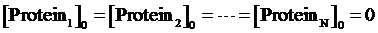Step 3: To make a cycle for each gene,Circuitn(n=1,...,N) , in the network. If Circuitn is regulated by Circuitm, we substitute the Circuitm’s concentration of targeted protein obtained from the former cycle into one of the following equations, (4)(6)(8)(9)(10)(11), in order to compute the regulated Circuitn’s concentration of targeted protein.

Step 4: If the relative error of ([Protein1]k,[Protein2]k,...,[ProteinN]k) and ([Protein1]k-1,[Protein2]k-1,...,[ProteinN]k-1) is less than a fixed threshold, or the number of iterations k is greater than a set limit, then please go back to step 3. Otherwise, please continue to step 5.

Step 5: To consider each genes’ concentration of targeted protein found in the last iteration as the equilibrium concentration. Therefore, we have [Protein1]s',[Protein2]s',...,[ProteinN]s'.

Moreover, with [Protein1]s,[Protein2]s,...,[ProteinN]s obtained from step 1, we can compute the regulation rate,.

Although this algorithm is just an approximation of regulation rate, the result obtained is highly consistent with simulation by numerical method and the accuracy would be increased after several times of iteration. Therefore, we may conclude this method is more effective and reliable.

## References

 Priscilla E. M. Purnick and Ron Weiss ,The second wave of synthetic biology: from modules to systems,Nature, 2009 ,10,410-422

 Barry Canton1, Anna Labno, Drew Endy, Measuring the activity of BioBrick promoters using an in vivo reference standard,Nature Biotechnolgy,2008,26(7),787-793

 Kelly JR.el, Measuring the activity of BioBrick promoters using an in vivo reference standard, J Biol Eng. 2009,3

 Iadevaia S., and Mantzaris N.V., Genetic network driven control of PHBV copolymer composition, Journal of Biotechnology, 2006, 122(1), 99-121

 Braun D., Basu S., and Weiss R., Parameter Estimation for Two Synthetic Gene Networks: A Case Study, IEEE Int Conf. Acoustics, Speech, and Signal Processing 2005, 5

 J. De Anda, A. Poteete, and R. Sauer, P22 c2 repressor:Domain structure and function, J. Biol. Chem. 1983,258(17)

 Alon, Uri. “An Introduction to Systems Biology Design Principles of Biological Circiuts.” London: Chapman & Hall/CRC, 2007

 Mario Andrea MarchisioA guide to composable parts and pools, ETHZ,2008

 MadsKaern, Timothy C. Elston, William J. Blakeand James J. Collins,STOCHASTICITY IN GENEEXPRESSION: FROM THEORIESTO PHENOTYPES, NATURE REVIEWS GENETICS，2005，451-464

 Gillespie, Approximate accelerated stochastic simulation of chemically reacting systems. Journal of Chemical Physics, 115:1716-1733

 Bor-Sen Chen and Yu-Te ChangA systematic molecular circuit design method for gene networksunder biochemical time delays and molecular noises, BMC Systems Biology 2008, 2:103

 T. Tian, K.Burrage, P. M. Burrage and M. Carletti,Stochastic Delay Differential Equations for Genetic Regulatory Networks, Special Issue of J. Comp and Applied Maths,2006

 Gregory Batt, BoyanYordanov, Ron Weissand CalinBeltaRobustness analysis and tuning of synthetic gene networks,BIOINFORMATICS, 2007,23 ,2415–2422